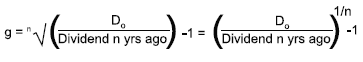Skip to main content# ACCAPEDIA

Kaplan Wiki > ACCAPEDIA > Wiki Pages > Cost of Equity

## # Cost of EquityFavorite|Discussions
| |History
|
 Incoming Links### Quick Launch View All Site Content#toc{width:623px;} div.addthis_toolbox{float:right;height:25px;margin:10px -16px 0px 0px;width:62px;} div.addthis_toolbox a{width:50px;overflow:hidden;margin-right:10px;} div.addthis_toolbox a.addthis_button_google_plusone{width:30px;} div.addthis_toolbox a.addthis_button_tweet{width:58px;} div.addthis_toolbox a.addthis_button_facebook_like{margin-right:0px;} .right-nav-col{float:right;min-height:500px;margin-top:-164px;margin-left:20px;margin-bottom:15px;width:300px;} .inner-nav{background:#F1F1F1;border:1px solid #B0B0B0;margin-top:47px;padding:2px;min-height:586px;width:296px;} .inner-nav h2, .inner-nav h3{background:#C0B9DA;border-bottom:none;display:block;padding:8px;text-align:center;width:279px;} .inner-nav h2{margin:0px;width:} .inner-nav h3{float:left;font-size:12px;margin-top:5px;margin-bottom:5px;} .inner-nav a{color:#1E0773;} .inner-nav p{text-align:center;margin:8px 0px;} h2{width:617px;} div.page-links{float:left;width:293px;text-align:center;white-space:no-wrap;} div.page-links span{padding:0px 8px;} div.page-links a{display:inline-block;margin-bottom:2px;} body #MSO_ContentTable {margin-top: -17px;}

# Cost of capital

Back up a level
 Contents [Hide]

# Cost of equity

The cost of equity is the return required by a company's shareholders and needs to be determined as part of calculating a weighted average cost of capital for use as a discount rate for investment appraisal.Ve and Vd are the market values of equity and debt respectively.

ke and kd are the returns required by the equity holders and the debt holders respectively.

T is the corporation tax rate.

ke is the cost of equity.

kd(1-T) is the post tax cost of debt.

## Using the Dividend Valuation Model to determine the cost of equity

The dividend valuation model states that:

The value of the company/share is the present value of the expected future dividends discounted at the shareholders' required rate of return.

Assuming a constant growth rate in dividends, g:

P0 = D0(1 + g) / (ke - g)

D 0 = current level of dividend

P 0 = current share price

g = estimated growth rate in dividends

If we need to derive ke the formula can be rearranged to:

ke = [D0 (1+g) / P0 ] + g

### Estimating growth

Two ways of estimating the likely growth rate of dividends are:

• extrapolating based on past dividend patterns
• assuming growth is dependent on the level of earnings retained in the business

Past dividends

This method assumes that the past pattern of dividends is a fair indicator of the future.

The formula for extrapolating growth can be written as:where:

n = number of years of dividend growth.

This method can only be used if:

• recent dividend pattern is considered typical
• historical pattern is expected to continue.

As a result, this method will usually only be appropriate to predict growth rates over the short term.

The earnings retention model (Gordon's growth model)

Assumption

• The higher the level of retentions in a business, the higher the potential growth rate.

The formula is therefore:

g = br

where:

r = post-tax accounting rate of return earned on reinvested funds

b = earnings retention rate.

Determining "r"

One way of determining r is to use the Accounting Rate of Return on equity calculated as:

r = PAT / opening shareholders' funds

The weakness of the ARR as a measure of return is that:

• it ignores the level of investment in intangible assets
• in the long run, the return on new investment tends to the cost of equity.

Hence, if a short term growth rate is required, the ARR provides a fair approximation for use in the growth model. However, if a long term growth rate is needed, ke should be used as the percentage return. To avoid a recursion problem, this should be derived using CAPM.

## Using CAPM to determine the cost of equity

The CAPM can be used to derive a required return as long as the systematic risk of an investment is known.

In the case of equity an equity beta can be used to derive a required return on equity as follows:

ke = Rf + βequity (E(Rm) - Rf)

If obtaining the βequity from a quoted company in the same industry sector, then it is likely that the proxy βequity will have to be degeared and regeared. Click here for further details on manipulating betas.

The CAPM model is based upon the assumption that investors are well diversified, so will have eliminated all the unsystematic (specific) risk from their portfolios. The beta factor is a measure of the level of systematic risk (general, market risk) faced by a well diversified investor.Created at 9/16/2012 4:09 PM  by System Account  (GMT) Greenwich Mean Time : Dublin, Edinburgh, Lisbon, London Last modified at 11/13/2012 11:40 AM  by System Account  (GMT) Greenwich Mean Time : Dublin, Edinburgh, Lisbon, London### Rating :Ratings & Comments  (Click the stars to rate the page)### Tags:### Recent Discussions

 There are no items to show in this view.ACCAPEDIA - Cost of Equity